# In each of the following figures, you find who triangles

Question:

In each of the following figures, you find who triangles. Indicate whether the triangles are similar. Give reasons in support of your answer.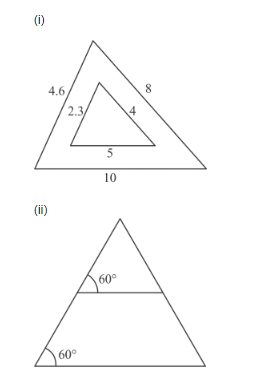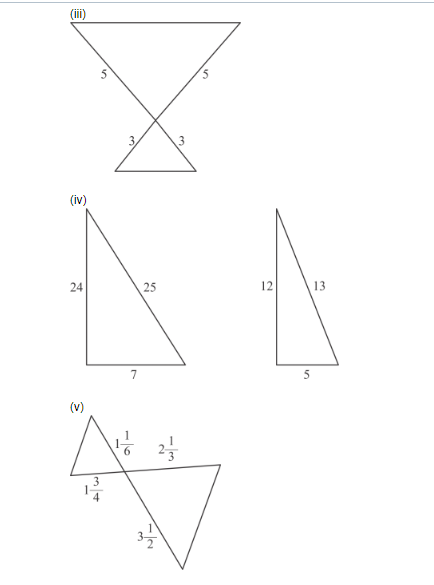Solution:

(i) In two triangles, we observe that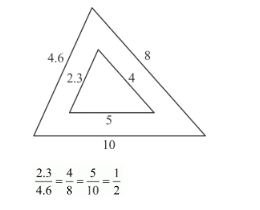In similar triangle corresponding sides are proportional to each other.

Therefore, by SSS-criterion of similarity,

yes two triangles are similar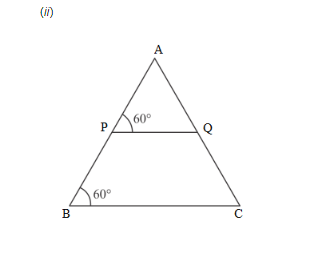PQ || BC             (Corresponding angles formed are equal)

In ΔAPQ and ΔABC,

$\angle A P Q=\angle B$ (Corresponding angles)

$\angle P A Q=\angle B A C$ (Common)

So, $\triangle \mathrm{APQ}-\Delta \mathrm{ABC}$ (A.A Similarity)

yes two triangles are similar

(iii) In two triangle, we observe that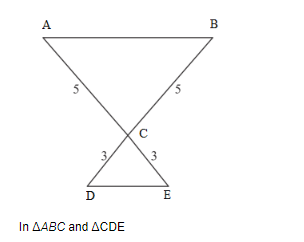$\frac{C D}{C E}=\frac{C B}{C A}$

$\angle A C B=\angle D C E$ (Vertically opposite angles)

$\triangle A B C-\triangle C D E$ (SAS Similarity)

yes two triangles are similar

(iv) In two triangle, we observe that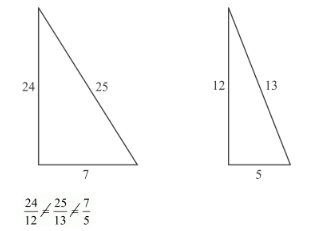In two triangles corresponding sides are not proportional to each other.

No two triangles are not similar.

(v) In two triangle, we observe that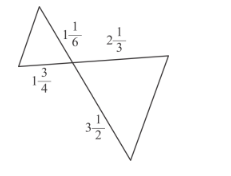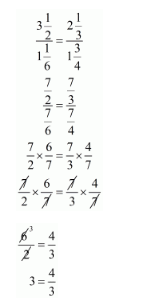In two triangles corresponding sides are not proportional to each other.

No two triangles are not similar.